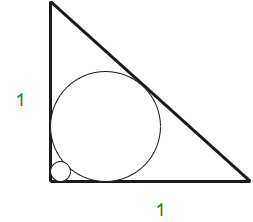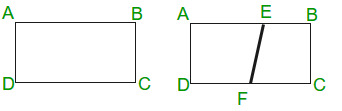Open In App

# Adobe Interview Experience | Set 23 (1 Year Experienced)

I recently interviewed at Adobe, Noida office and got the offer. I had 1-year work experience.

It was a standard process comprising of an aptitude and technical test on Hackerrank. It’s the same every time. You can have a look at the test in previous archives.

• Screening
• Aptitude Test
• Technical Test having 13 MCQs and 7 standard coding questions.

Interviews at Noida Office

• Round 1.
The interview started with my general introduction. The interviewer wanted to know if I had in depth knowledge of what I am working on, so he asked all sorts of questions related to the technologies and why they were used and not something else.

1. Questions Related to C, C++ – How are .h files loaded and linked with their .c files. I did not know much about it as I had experience in Java and told him the equivalent answer in Java.
2. How to find the minimum element in a rotated array? e.g. 4 5 6 1 2 3
Gave the answer using Binary Search

3. How to find a common node between two Linked Lists?
```1→2→3→4→NULL
|
5→6```

Gave an answer using the difference in length of LLs and wrote the code which handled all the cases.

4. Then he discussed Operating System Concepts, Virtual Memory, Paging, etc.

Note. Make sure you write code which handles all the test cases especially the boundary ones.

• Round 2.
The interview started with my brief introduction. This time the interview was more interested in my hobbies and passions. He asked me what do you do to keep yourself updated with the latest technology trends?

1. After that he asked me only one question related to geometry. The question was given an isosceles right angled triangle. Find the radius of the smaller circle.Solved it using basic pythagoras theorem.

It was weird that he asked only one question.

• Round 3

This round was a tricky one.

The interviewer asked me what all had been asked to me till now. I confidently said that I liked that goemetry was asked. I shouldn’t have said that… 😛

1. The question was given a rectangle ABCD with length l and breadth b. Now it is folded along diagonal BD. i.e. A is joined to C. Find the length of the line segment EFSolved the question with his hints. Basically it involved some deductions related to imagination and pythagoras theorem. Basically AE = EC and EF = EC. After that it is simple geometry question.

2. How do you heapify an array? What is the time complexity involved? Apparently, he was interested in the exact complexity. I told him that we always start heapifying from the last parent whose index in the array is (n-1)/2.
3. He then asked me about how we heapify individual elements, etc. and how heap sort works.

Basically, he wanted me to answer that we do not heapify elements from (n-1)/2 till n which decreases the time complexity from nlogn which I answered in the end.

4. ```int a = 8;
char *b = (int *) a;```

Where does b point to?

Now this question was related to Big endian and little endian as in he wanted me to ask if the architechture is little endian or big endian. I told him I did not know about this.

After this question, I told him that I am from Mechanical background and I did not know much about computer architecture. He was a little shocked and skipped the OS questions. Lucky me!!!

5. How to know if a number is a power of 2 in O(1)? n&(n – 1)== 0?true:false
6. Given an array of numbers all of which are repeated only one non-repeated, find the non repeated number.
7. Gave the algorithm using XOR.

8. Then he extended the previous question to find if two numbers are non repeated. He wanted the XOR approach again.
9. Given two binary trees A and B. Check if B is a subtree of A. Solved using normal tree traversal.
10. Find the kth smallest element in an unsorted array of numbers.

First gave the approach using Heap. Put all the elements of an array in a min heap and extract k times. But he was interested in a better approach.

Answered using a quick select algorithm (modified quicksort).

He told me there is a more optimized approach to the selection of pivot elements using order statistics. I did not know the order statistics method but he was quite satisfied with the basic quick select algorithm.

• Round 4: Director Round
1. My brief introduction. Why low CGPA, etc. ?
2. Given an array of size m out of which n indexes are allocated. How do you minimize the number of comparisons when you search an element in this array?
3. I wrote basic linear search code. Then he asked me how do you minimize the number of comparisons.

4. Given n points in a plane which form a polygon. Find if a random point exists inside that polygon or not?
5. How would you find the volume of a lake. He was interested in my approach. I gave 2-3 methods, one of which was quite funny on which he laughed at me.

In the end he asked me if I had any questions regarding the role and all.

I would like to take a moment and thank GeeksforGeeks for helping me in my interview preparation. Cheers to you guys!!!

If you like GeeksforGeeks and would like to contribute, you can also write an article and mail your article to contribute@geeksforgeeks.org. See your article appearing on the GeeksforGeeks main page and help other Geeks.